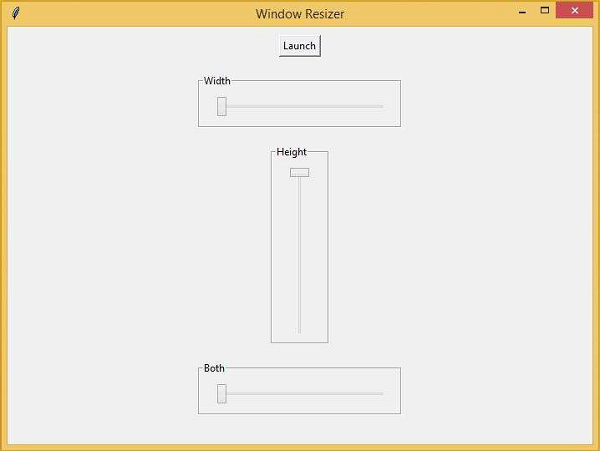# Window Resizer Control Panel in Tkinter

PythonTkinterGUI-Programming

In this article, we will create a GUI-based window resizer control panel that will have a pane to resize the window by its height or width.

In order to create the application, we will first create a slider that will help to resize the window size. The sliders are available in the ttk library of tkinter. We will import “ttk” first. Then, we will launch a new window which needs to be resized.

Let us first import all the required libraries in the notebook and design the control bars using sliders.

## Example

# Import the required Libraries
from tkinter import *
from tkinter import ttk

# Create Object
win = Tk()

# Set title
win.title("Window Resizer")
lab= Label(win, text="Window Resizer", font=('Poppins bold', 20))

#Define the geometry for the window or frame
win.geometry("500x500")

# Create a button to launch a new window
launch_button = Button(win,text = "Launch")

# Add Label Frames for width, height and both
width_frame = LabelFrame(win, text = "Width")

height_frame = LabelFrame(win, text = "Height")

both_frame = LabelFrame(win, text = "Both")

#Width Slider
width_slider = ttk.Scale(width_frame,from_ = 100,to = 500,orient = HORIZONTAL,length = 200, value = 100)

#Height Slider
height_slider = ttk.Scale(height_frame, from_ = 100, to = 500, orient = VERTICAL,length = 200, value = 100)

#Both Slider
both_slider = ttk.Scale(both_frame, from_ = 100,to = 500, orient = HORIZONTAL,length = 200, value = 100)

# Keep running the window
win.mainloop()

After creating the GUI for Sliders and controls, we will define the different functions that will be invoked in the sliders and window controls.

First, we will create a function for opening a new window where the control movement will appear. Then, we will define the function for width, height, and both.

After defining the function, it will be as follows −

## Example

# Import the required Libraries
from tkinter import *
from tkinter import ttk

# Create Object
win = Tk()

# Set title
win.title("Window Resizer")
lab= Label(win, text="Window Resizer", font=('Poppins bold', 20))

#Define the geometry for the window or frame
win.geometry("500x500")

#Define Functions for all different events
# Open New Window
def launch_win():
global win1
win1 = Toplevel()
win1.geometry("100x100")

# Change width
def change_width(x):
win1.geometry(f"{int(width_slider.get())}x{int(height_slider.get())}")

#Change height
def change_height(x):
win1.geometry(f"{int(width_slider.get())}x{int(height_slider.get())}")

#Change both width and height
def change_both(x):
win1.geometry(f"{int(both_slider.get())}x{int(both_slider.get())}")

# Create a button to launch a new window
launch_button = Button(win,text = "Launch", command= launch_win)

# Add Label Frames for width, height and both
width_frame = LabelFrame(win, text = "Width")

height_frame = LabelFrame(win, text = "Height")

both_frame = LabelFrame(win, text = "Both")

#Width Slider
width_slider = ttk.Scale(width_frame,from_ = 100,to = 500,orient =
HORIZONTAL,length = 200, command= change_height, value=100)

#Height Slider
height_slider = ttk.Scale(height_frame, from_ = 100, to = 500, orient =
VERTICAL,length = 200,command= change_width, value=100)

#Both Slider
both_slider = ttk.Scale(both_frame, from_ = 100,to = 500, orient =
HORIZONTAL,length = 200,command= change_both, value=100)
win.mainloop()